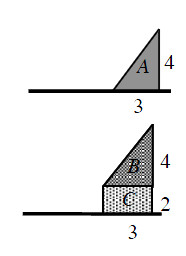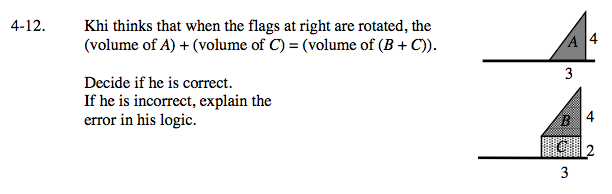### Home > CALC > Chapter 4 > Lesson 4.1.1 > Problem4-12

4-12.

Khi thinks that when the flags below are rotated, the (volume of A) + (volume of C) = (volume of (B + C)).Decide if he is correct. If he is incorrect, explain the error in his logic. Homework Help ✎Start by visualizing the terms in Khi's equation: What does Volume A and Volume C look like? What does Volume (B + C) look like?

Volume A is cone with radius 4. Volume C is a cylinder with radius 2. Each is a separate 3D solid whose volumes are added together after they are computed: V = [cone with r = 4] + [cylinder with r = 2]

Try computing the volume of each:

Volume A + Volume C = cone + cylinder

Volume (B + C) = BigCone − SmallCone

Evaluate and compare.

Volume (B + C) is a single 3D solid that looks like a cone with its top cut off. To compute its volume, it must be dissected into pieces:
V = [large cone with r = 6] − [small cone with r = 2]

Use the eTool below to visualize this problem.
Click on the link to the right to view the full version of the eTool: Calc 4-12 eTool